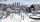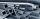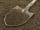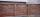# Prism + body volume - examples - page 3

1. PrismFind the volume and surface area of prism with base of an equilateral triangle with side 7 dm long and the body height of 1.5 m.
2. Ice and waterWe want to cover rectangular rink with dimensions of 55 m and 25 m with 4cm thick layer of ice. How many liters of water we need if after freezing water increases its volume by 10%?
3. RainfallOn Thursday, fell 1 cm rainfall. How many liters of water fell to rectangular garden with dimensions of 22 m x 35 m?
4. Water channelThe cross section of the water channel is a trapezoid. The width of the bottom is 19.7 m, the water surface width is 28.5 m, the side walls have a slope of 67°30' and 61°15'. Calculate how much water flows through the channel in 5 minutes if the water flow
5. Quadrangular prismCalculate the volume and surface area of a regular quadrangular prism 35 cm high and the base diagonal of 22 cm.
6. 3s prismIt is given a regular perpendicular triangular prism with a height 19.0 cm and a base edge length 7.1 cm. Calculate the volume of the prism.
7. Four sided prismCalculate the volume and surface area of a regular quadrangular prism whose height is 28.6cm and the body diagonal forms a 50 degree angle with the base plane.
8. The factoryThe factory ordered 500 hexagonal steel bars of square section with 25 mm side. How many cars with a load capacity of 3 tonnes will be needed for bars move if the steel density is 7,850 kg.m-3?
9. Prism - eq triangleCalculate the volume and surface of the prism with the base of an equilateral triangle with side a = 4cm and the body height is 6cm.
10. Wood lumberWooden lumber is 4 m long and has a cross section square with side 15 cm. Calculate: a) the volume of lumber b) the weight of the lumber if 1 m3 weighs 790 kg
11. ClayHow many cubic centimeters of clay is in a pit of dimensions 4 m x 3 m x 3 m?
12. Cuboid edges in ratioCuboid edges lengths are in ratio 2:4:6. Calculate their lengths if you know that the cuboid volume is 24576 cm3.
13. Brick wallGarden 70 m long and 48 m wide should surround with wall 2.1 meters high and 30 cm thick. Wall will be built on the garden ground. How many will we need bricks if to 1 m³ is required approximately 300 bricks?
14. TrenchThe trench is a four-sided prism. The cross section has a trapezoidal shape with basements of 4m and 6m, the length of the trench is 30m. What is the depth of the trench if we dig 60,000 l of soil.
15. Triangular prismThe perpendicular triangular prism is a right triangle with a 5 cm leg. The content of the largest wall of the prism is 130 cm2 and the body height is 10 cm. Calculate the body volume.
16. Surface of cubesPeter molded a cuboid 2 cm, 4cm, 9cm of plasticine. Then the plasticine split into two parts in a ratio 1:8. From each part made a cube. In what ratio are the surfaces of these cubes?
17. Cubes into cuboidHow many 12 centimeter cubes fit into the block (cuboid) with 6dm, 8,4dm and 4,8?
18. Water blockA block with a 50 cm2 base is filled with water 5 cm under the edge. How many can sugar cubes with 2 cm edge be thrown into a container that overflow water?
19. Scale factorA prism with a volume of 1458 mm3 is scaled down to a volume of 16mm3. What is the scale factor in fraction form?
20. Triangular prismCalculate the volume of a triangular prism 10 cm high, the base of which is an equilateral triangle with dimensions a = 5 cm and height va = 4,3 cm

Do you have an interesting mathematical example that you can't solve it? Enter it, and we can try to solve it.

To this e-mail address, we will reply solution; solved examples are also published here. Please enter e-mail correctly and check whether you don't have a full mailbox.